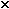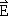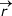# Problem: You want to create an electric field  = &lt; 0, 5.  104, 0&gt; N/C at location &lt; 0, 0, 0&gt;.A. Where would you place a proton to produce this field at the origin?p =  mB. Instead of a proton, where would you place an electron to produce this field at the origin?e =  m(Hint: This problem will be much easier if you draw a diagram.)

###### FREE Expert Solution

The electric field is expressed as:

$\overline{){\mathbf{E}}{\mathbf{=}}\frac{\mathbf{k}\mathbf{q}}{{\mathbf{r}}^{\mathbf{2}}}}$, where E is the electric field, k is Coulomb's constant, q is the charge, and r is the distance.

A.

The electric field, E = < 0, 5.104, 0> N/C  is in the y-direction only.

This means that the electric field in the x-direction and z-direction is zero.

The charged particle will be placed only on the y-axis.

84% (404 ratings)###### Problem Details

You want to create an electric field= < 0, 5.104, 0> N/C at location < 0, 0, 0>.

A. Where would you place a proton to produce this field at the origin?p =  m

B. Instead of a proton, where would you place an electron to produce this field at the origin?e =  m

(Hint: This problem will be much easier if you draw a diagram.)